## A sample of chlorine gas occupies a volume of 0.025L at 300 K. At 600K, what will the volume be? A)808 L B)50 L C)0.050 L

Question

A sample of chlorine gas occupies a volume of 0.025L at 300 K. At 600K, what will the volume be?
A)808 L
B)50 L
C)0.050 L
D)0.088 L

in progress 0
5 months 2021-08-16T04:47:30+00:00 1 Answers 2 views 0

1. Answer:  The volume at 600 K will be 0.050 L.

Explanation:

Given: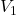= 0.025 L,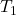= 300 K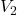= ?,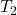= 600 K

Formula used is as follows.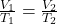Substitute the values into above formula as follows.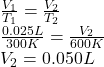Thus, we can conclude that the volume at 600 K will be 0.050 L.